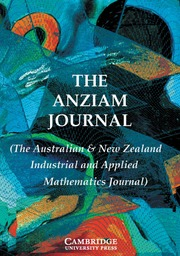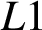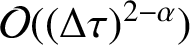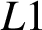Home
Hostname: page-component-7f7b94f6bd-98q29 Total loading time: 2.254 Render date: 2022-06-29T13:46:03.404Z Has data issue: true Feature Flags: { "shouldUseShareProductTool": true, "shouldUseHypothesis": true, "isUnsiloEnabled": true, "useRatesEcommerce": false, "useNewApi": true } hasContentIssue trueThe ANZIAM Journal

## Abstract

We propose a Legendre–Laguerre spectral approximation to price the European and double barrier options in the time-fractional framework. By choosing an appropriate basis function, the spectral discretization is used for the approximation of the spatial derivatives of the time-fractional Black–Scholes equation. For the time discretization, we consider the popular$L1$ finite difference approximation, which converges with order$\mathcal {O}((\Delta \tau )^{2-\alpha })$ for functions which are twice continuously differentiable. However, when using the$L1$ scheme for problems with nonsmooth initial data, only the first-order accuracy in time is achieved. This low-order accuracy is also observed when solving the time-fractional Black–Scholes European and barrier option pricing problems for which the payoffs are all nonsmooth. To increase the temporal convergence rate, we therefore consider a Richardson extrapolation method, which when combined with the spectral approximation in space, exhibits higher order convergence such that high accuracies over the whole discretization grid are obtained. Compared with the traditional finite difference scheme, numerical examples clearly indicate that the spectral approximation converges exponentially over a small number of grid points. Also, as demonstrated, such high accuracies can be achieved in much fewer time steps using the extrapolation approach.

## MSC classification

Type
Research Article
Information
The ANZIAM Journal , April 2021 , pp. 228 - 248

## Access options

Get access to the full version of this content by using one of the access options below. (Log in options will check for institutional or personal access. Content may require purchase if you do not have access.)

## References

Black, F. and Scholes, M., “The pricing of options and other corporate liabilities”, J. Polit. Econ. 81 (1973) 637654, available at https://www.jstor.org/stable/1831029.CrossRefGoogle Scholar
Cen, Z., Huang, J., Xu, A. and Le, A., “Numerical approximation of a time-fractional Black–Scholes equation”, Comput. Math. Appl. 75 (2018) 28742887; doi:10.1016/j.camwa.2018.01.016.CrossRefGoogle Scholar
Chen, C., Wang, Z. and Yang, Y., “A new operator splitting method for American options under fractional Black–Scholes models”, Comput. Math. Appl. 77 (2019) 21302144; doi:10.1016/j.camwa.2018.12.007.CrossRefGoogle Scholar
Chen, H., , S. and Chen, W., “Finite difference/spectral approximations for the distributed order time fractional reaction-diffusion equation on an unbounded domain”, J. Comput. Phys. 315 (2016) 8497; doi:10.1016/j.jcp.2016.03.044.CrossRefGoogle Scholar
Chen, H., , S. and Chen, W., “Spectral and pseudospectral approximations for the time fractional diffusion equation on an unbounded domain”, J. Comput. Appl. Math. 304 (2016) 4356; doi:10.1016/j.cam.2016.03.010.CrossRefGoogle Scholar
Chen, W. and Wang, S., “A 2nd-order ADI finite difference method for a 2D fractional Black–Scholes equation governing European two asset option pricing”, Math. Comput. Simulation 171 (2020) 279293; doi:10.1016/j.matcom.2019.10.016.CrossRefGoogle Scholar
Chen, W., Xu, X. and Zhu, S., “Analytically pricing European-style options under the modified Black–Scholes equation with a spatial-fractional derivative”, Quart. Appl. Math. 72 (2014) 597611, available at https://www.jstor.org/stable/43639126.CrossRefGoogle Scholar
Chen, W., Xu, X. and Zhu, S. P., “A predictor-corrector approach for pricing American options under the finite moment log-stable model”, Appl. Numer. Math. 97 (2015) 1529; doi:10.1016/j.apnum.2015.06.004.CrossRefGoogle Scholar
Chen, W., Xu, X. and Zhu, S. P., “Analytically pricing double barrier options based on a time-fractional Black–Scholes equation”, Comput. Math. Appl. 69 (2015) 14071419; doi:10.1016/j.camwa.2015.03.025.CrossRefGoogle Scholar
Chen, X., Ding, D., Lei, S-L. and Wang, W., “A fast preconditioned iterative method for two-dimensional options pricing under fractional differential models”, Comput. Math. Appl. 79 (2020) 440456; doi:10.1016/j.camwa.2019.07.010.CrossRefGoogle Scholar
Crank, J. and Nicolson, P., “A practical method for numerical evaluation of solutions of partial differential equations of the heat conduction type”, Math. Proc. Cambridge Philos. Soc. 43 (1947) 5067; doi: 10.1017/S0305004100023197.CrossRefGoogle Scholar
De Staelen, R. H. and Hendy, A. S., “Numerically pricing double barrier options in a time-fractional Black–Scholes model”. Comput. Math. Appl. 74 (2017) 11661175; doi:10.1016/j.camwa.2017.06.005.CrossRefGoogle Scholar
Diethelm, K. and Walz, G., “Numerical solution of fractional order differential equations by extrapolation”, Numer. Algorithms 16 (1997) 231253; doi:10.1023/A:1019147432240.CrossRefGoogle Scholar
Farhadi, A., Salehi, M. and Erjaee, G. H., “A new version of Black–Scholes equation presented by time-fractional derivative”, Iran J. Sci. Technol. Trans. A Sci. 42 (2018) 21592166; doi:10.1007/s40995-017-0244-7.CrossRefGoogle Scholar
Feng, L. and Linetsky, V., “Pricing options in jump-diffusion models: an extrapolation approach”, Oper. Res. 56 (2008) 304325, available at http://www.jstor.org/stable/25147189.CrossRefGoogle Scholar
Golbabai, A., Nikan, O. and Nikazad, T., “Numerical analysis of time fractional Black–Scholes European option pricing model arising in financial market”, Comput. Appl. Math. 38 (2019) 173; doi:10.1007/s40314-019-0957-7.CrossRefGoogle Scholar
Huang, J., Cen, Z. and Zhao, J., “An adaptive moving mesh method for a time-fractional Black–Scholes equation”, Adv. Difference Equ. 2019 (2019) 516; doi:10.1186/s13662-019-2453-1.CrossRefGoogle Scholar
Jin, B., Lazarov, R. and Zhou, Z., “An analysis of the$\mathrm{L}1$ scheme for the subdiffusion equation with nonsmooth data”, IMA J. Numer. Anal. 36 (2016) 197221; doi:10.1093/imanum/dru063. Google Scholar
Jumarie, G., “Derivation and solutions of some fractional Black–Scholes equations in coarse-grained space and time. Application to Merton’s optimal portfolio”, Comput. Math. Appl. 59 (2010) 11421164; doi:10.1016/j.camwa.2009.05.015.CrossRefGoogle Scholar
Koleva, M. and Vulkov, L., “Numerical solution of time-fractional Black–Scholes equation”, Comput. Appl. Math. 36 (2017) 16991715; doi:10.1007/s40314-016-0330-z.CrossRefGoogle Scholar
Lin, Y., Li, X. and Xu, C., “Finite difference/spectral approximations for the fractional cable equation”, Math. Comp. 80 (2011) 13691396; doi:10.1090/S0025-5718-2010-02438-X.CrossRefGoogle Scholar
Lin, Y. and Xu, C., “Finite difference/spectral approximations for the time-fractional diffusion equation”, J. Comput. Phys. 225 (2007) 15331552; doi:10.1016/j.jcp.2007.02.001.CrossRefGoogle Scholar
Mohammadi, R., “Quintic B-spline collocation approach for solving generalized Black–Scholes equation governing option pricing”, Comput. Math. Appl. 69 (2015) 777797; doi:10.1016/j.camwa.2015.02.018.CrossRefGoogle Scholar
Pal, K., Liu, F. and Yan, Y., “Numerical solutions for fractional differential equations by extrapolation”, in: Finite Difference Methods, Theory and Applications. FDM 2014, Volume 9045 of Lect. Notes Comp. Sci. (eds Dimov, I., Faragó, I. and Vulkov, L.), (Springer, Cham, 2015) 299306; https://link.springer.com/chapter/10.1007%2F978-3-319-20239-6_32.CrossRefGoogle Scholar
Prathumwan, D. and Trachoo, K., “On the solution of two-dimensional fractional Black–Scholes equation for European put option”, Adv. Difference Equ. 2020 (2020) 146; doi:10.1186/s13662-020-02554-8.CrossRefGoogle Scholar
Quarteroni, A., Numerical Models for Differential Problems, 2nd edn, Volume 8 of Modeling, Simulation and Applications (Springer, Milano, Italy, 2014); doi:10.1007/978-88-470-5522-3.Google Scholar
Roul, P., “A high accuracy numerical method and its convergence for time-fractional Black–Scholes equation governing European options”, Appl. Numer. Math. 151 (2020) 472493; doi:10.1016/j.apnum.2019.11.004.CrossRefGoogle Scholar
Roul, P., “A fourth order numerical method based on B-spline functions for pricing Asian options”, Comput. Math. Appl. 80 (2020) 504521; doi:10.1016/j.camwa.2020.04.001.CrossRefGoogle Scholar
Roul, P. and Goura, V. M. K. P., “A sixth order numerical method and its convergence for generalised Black–Scholes PDE”, J. Comput. Appl. Math. 377 (2020) 112881; doi:10.1016/j.cam.2020.112881.CrossRefGoogle Scholar
Shen, J., Tang, T. and Wang, L.-L., Spectral Methods. Algorithms, Analysis and Applications, Volume 41 of Springer Ser. Comput. Math. (eds. Bank, R., Graham, R. L., Stoer, J., Varga, R. and Yserentant, H.), (Springer, Berlin–Heidelberg, 2011); doi:10.1007/978-3-540-71041-7.Google Scholar
Song, L. and Wang, W., “Solution of the fractional Black–Scholes option pricing model by finite difference method”. Abstr. Appl. Anal. 2013 (2013) 194286; doi:10.1155/2013/194286.CrossRefGoogle Scholar
Wyss, W., “The fractional Black–Scholes equation”, Fract. Calc. Appl. Anal. 3 (2000) 5161.Google Scholar
Xing, Y. and Yan, Y., “A higher order numerical method for time fractional partial differential equations with nonsmooth data”, J. Comput. Phys. 357 (2018) 305323; doi:10.1016/j.jcp.2017.12.035.CrossRefGoogle Scholar
Yan, Y., Khan, M. and Ford, N. J., “An analysis of the modified$\mathrm{L}1$ scheme for time-fractional partial differential equations with nonsmooth data”, SIAM J. Numer. Anal. 56 (2018) 210227; doi:10.1137/16M1094257.CrossRefGoogle Scholar
Yan, Y., Pal, K. and Ford, N. J., “Higher order numerical methods for solving fractional differential equations”, BIT 54 (2014) 555584; doi:10.1007/s10543-013-0443-3.CrossRefGoogle Scholar
Yue, T., “Spectral element method for pricing European options and their Greeks”, Dissertation, Duke University, 2012, available at https://hdl.handle.net/10161/6156.Google Scholar
Zhang, H., Liu, F., Chen, S., Anh, V. and Chen, J., “Fast numerical simulation of a new time-space fractional option pricing model governing European call option”, Appl. Math. Comput. 339 (2018) 186198; doi:10.1016/j.amc.2018.06.030.Google Scholar
Zhang, H., Liu, F., Turner, I. and Yang, Q., “Numerical solution of the time fractional Black–Scholes model governing European options”, Comput. Math. Appl. 71 (2016) 17721783; doi:10.1016/j.camwa.2016.02.007.CrossRefGoogle Scholar

# Save article to Kindle

Note you can select to save to either the @free.kindle.com or @kindle.com variations. ‘@free.kindle.com’ emails are free but can only be saved to your device when it is connected to wi-fi. ‘@kindle.com’ emails can be delivered even when you are not connected to wi-fi, but note that service fees apply.

Find out more about the Kindle Personal Document Service.

SPECTRALLY ACCURATE OPTION PRICING UNDER THE TIME-FRACTIONAL BLACK–SCHOLES MODEL
Available formats
×

# Save article to Dropbox

To save this article to your Dropbox account, please select one or more formats and confirm that you agree to abide by our usage policies. If this is the first time you used this feature, you will be asked to authorise Cambridge Core to connect with your Dropbox account. Find out more about saving content to Dropbox.

SPECTRALLY ACCURATE OPTION PRICING UNDER THE TIME-FRACTIONAL BLACK–SCHOLES MODEL
Available formats
×

# Save article to Google Drive

To save this article to your Google Drive account, please select one or more formats and confirm that you agree to abide by our usage policies. If this is the first time you used this feature, you will be asked to authorise Cambridge Core to connect with your Google Drive account. Find out more about saving content to Google Drive.

SPECTRALLY ACCURATE OPTION PRICING UNDER THE TIME-FRACTIONAL BLACK–SCHOLES MODEL
Available formats
×
×